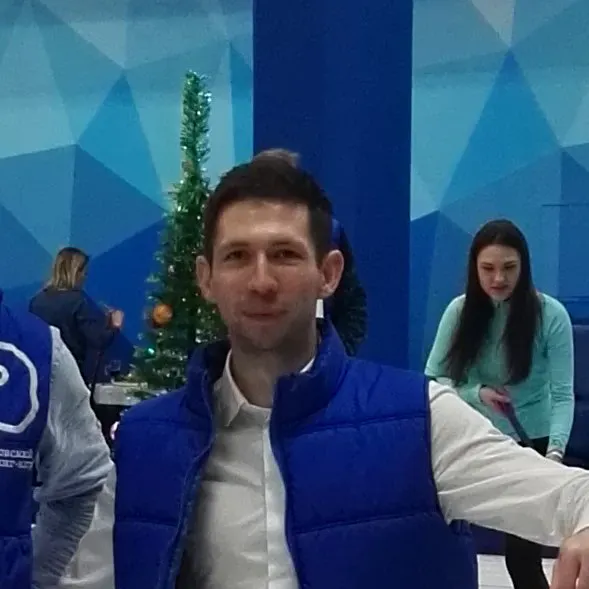# Air Surfer

It is a device that measures CO2 levels, inside and outside temperatures, and humidity.

IntermediateFull instructions provided39,598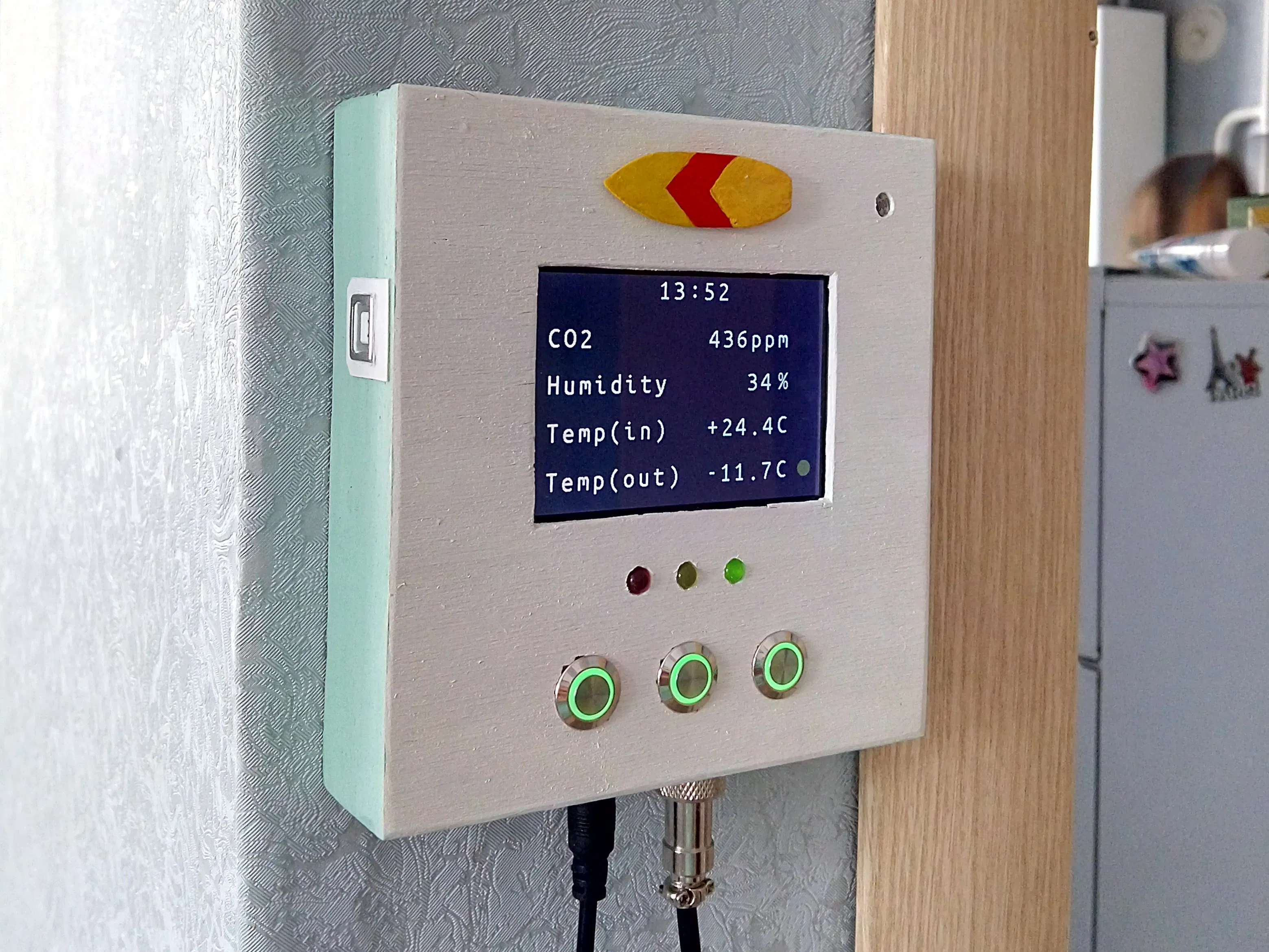## Things used in this project

### Hardware components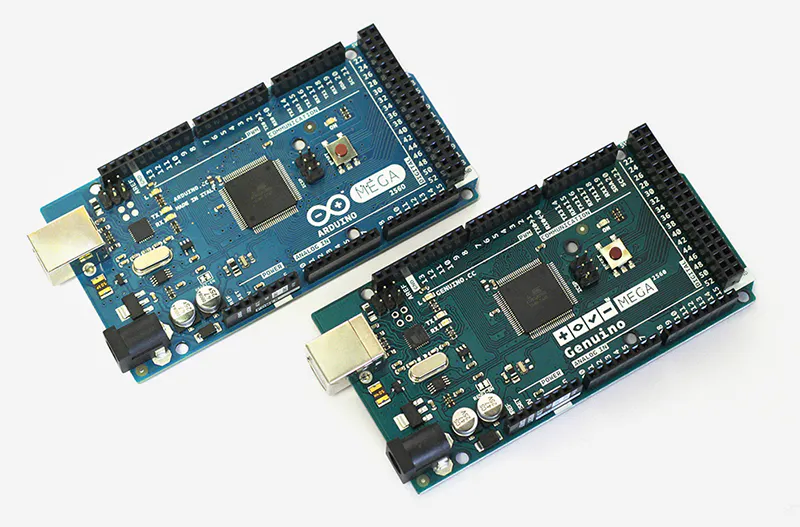Arduino Mega 2560 & Genuino Mega 2560
×1
 3.2 inch tft module for Mega 2560
×1
 Temperature probe
×1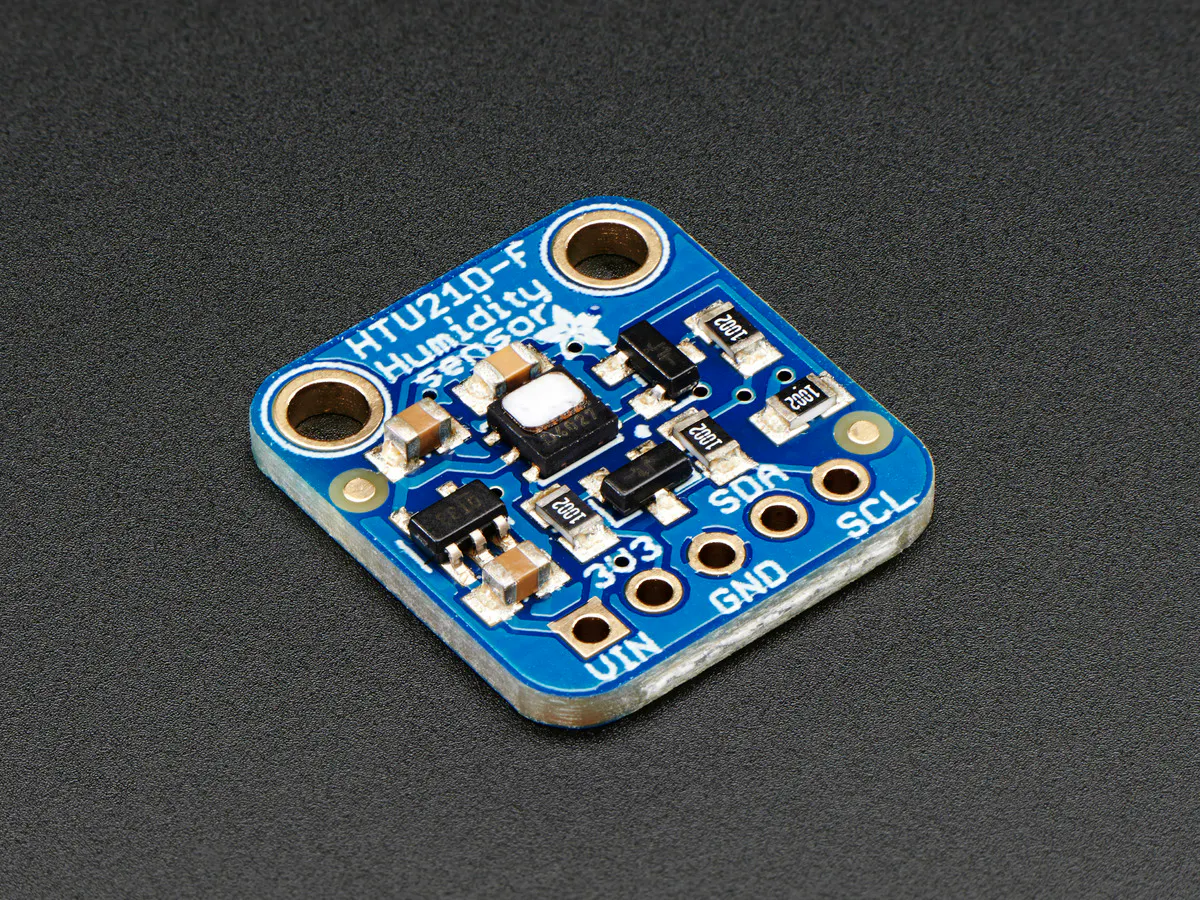×1
 mh-z19 CO2 sensor
×1
 RTC module
×1
×1
 Aviation plug L89 G12
×1

### Software apps and online servicesArduino IDE

## Schematics

### Basic connections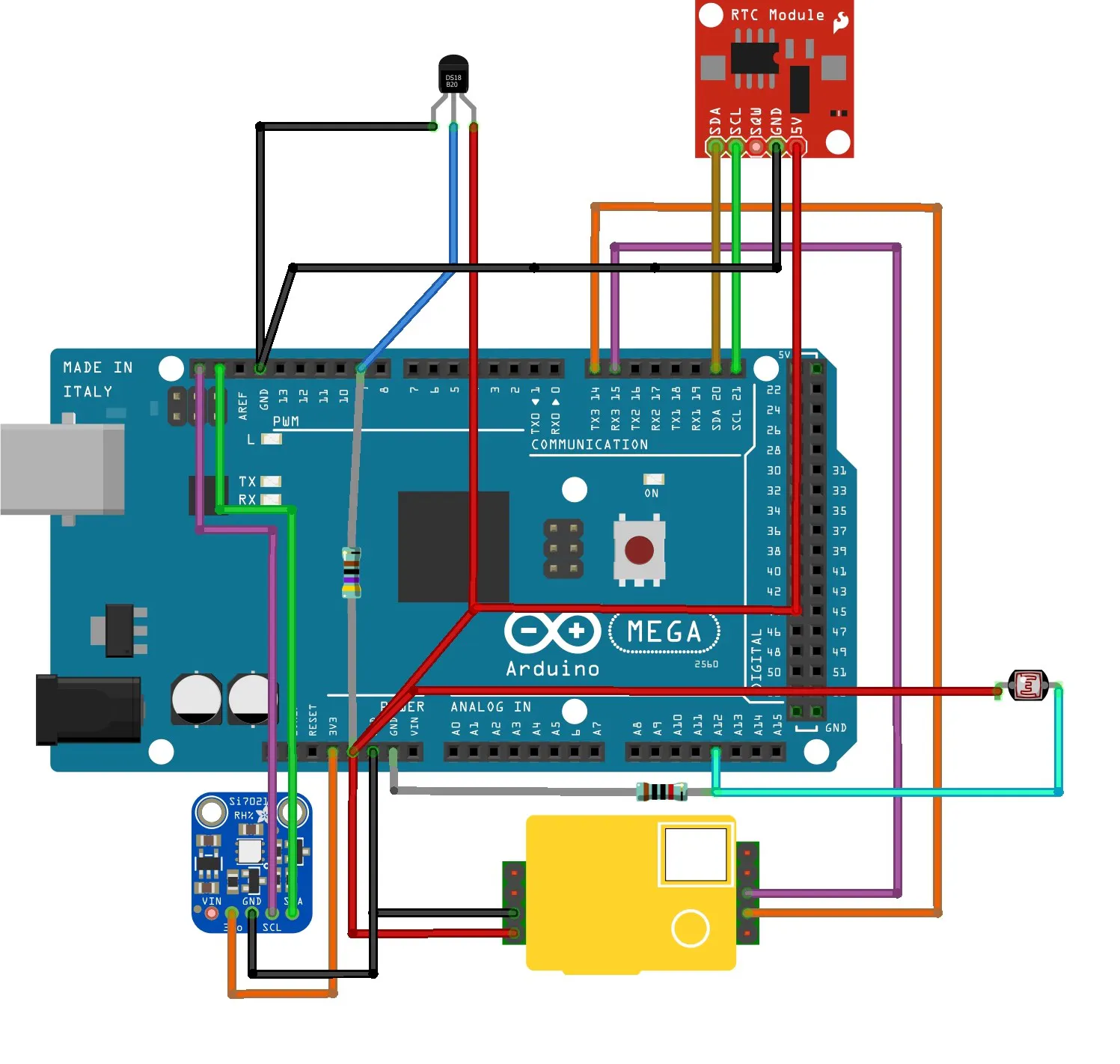## Code

### Air surfer code

Arduino
```#include <Wire.h>
#include "RTClib.h"
//#include <Arduino.h>
#include <SPI.h>
#include <SoftwareSerial.h>
#include <SparkFunHTU21D.h>
#include <OneWire.h>
#include <DallasTemperature.h>
#include <UTFT.h>

#define ONE_WIRE_BUS 9
#define GREENLED 5
#define YELLOWLED 7
#define REDLED 8
#define BUTTONLED 11

OneWire oneWire(ONE_WIRE_BUS);
DallasTemperature sensors(&oneWire);

UTFT myGLCD(CTE32HR, 38, 39, 40, 41);

HTU21D myHumidity;
RTC_DS1307 rtc;
extern uint8_t Ubuntu[];

byte cmd = {0xFF,0x01,0x86,0x00,0x00,0x00,0x00,0x00,0x79};
unsigned char response;
unsigned int ppm;
unsigned long time;

int hourupg;
int minupg;

float humd;
float temp;
int tcolor;
int Z; //LED brightness
boolean Reset = false; // for reseting display;

void lamps(void) {

//CO2
if (ppm<800){
myGLCD.setColor(255,140,255); //green
myGLCD.fillCircle(450, 87, 12);
analogWrite(GREENLED, Z);
analogWrite(YELLOWLED, 0);
analogWrite(REDLED, 0);
}
if (ppm>800 & ppm<1200) {
myGLCD.setColor(70,70,255); //Yellow
myGLCD.fillCircle(450, 87, 12);
analogWrite(GREENLED, 0);
analogWrite(YELLOWLED, Z);
analogWrite(REDLED, 0);
}
if (ppm>1200){
myGLCD.setColor(VGA_AQUA);
myGLCD.fillCircle(450, 87, 12);
analogWrite(GREENLED, 0);
analogWrite(YELLOWLED, 0);
analogWrite(REDLED, Z);
}

//Hum
if (humd>30 & humd<50)  {
myGLCD.setColor(255,140,255); //green
myGLCD.fillCircle(450, 149, 12);
}
if (humd>20 & humd<30)  {
myGLCD.setColor(70,70,255); //Yellow
myGLCD.fillCircle(450, 149, 12);
}
if (humd<20)  {
myGLCD.setColor(70,70,255); //Yellow
myGLCD.fillCircle(450, 149, 12);
}
if (humd>50 & humd<60)  {
myGLCD.setColor(70,70,255); //Yellow
myGLCD.fillCircle(450, 149, 12);
}
if (humd>60)  {
myGLCD.setColor(VGA_AQUA);
myGLCD.fillCircle(450, 149, 12);
}

//Temp ins

if (temp>20 & temp<27) {
myGLCD.setColor(255,140,255); //green
myGLCD.fillCircle(450, 213, 12);
} else {
myGLCD.setColor(70,70,255); //Yellow
myGLCD.fillCircle(450, 213, 12);
}
//Tempouts

if (sensors.getTempCByIndex(0)<10) {
myGLCD.setColor(70,70,255); //Yellow
myGLCD.fillCircle(450, 278, 12);
} else {
myGLCD.setColor(255,140,255); //green
myGLCD.fillCircle(450, 278, 12);
}}

void drawmain(void) {
myGLCD.setFont(Ubuntu);
if (tcolor==0) myGLCD.setColor(VGA_BLACK);
if (tcolor==1) myGLCD.setColor(VGA_SILVER);
if (tcolor==2) myGLCD.setColor(250,10,250);
if (tcolor==3) myGLCD.setColor(200,100,200);
if (tcolor==4) myGLCD.setColor(100,200,200);
if (tcolor==5) myGLCD.setColor(10,200,200);
if (tcolor==6) myGLCD.setColor(200,200,100);
if (tcolor==7) myGLCD.setColor(250,250,10);
if (tcolor==8) myGLCD.setColor(10,10,250);
if (tcolor==9) myGLCD.setColor(10,250,10);
if (tcolor==10) myGLCD.setColor(250,10,10);
if (tcolor==11) myGLCD.setColor(VGA_TEAL);
if (tcolor==12) myGLCD.setColor(VGA_AQUA);
DateTime now = rtc.now();
// Time
if (now.hour()<10)
{
myGLCD.print("0", 190, 7);
myGLCD.printNumI(now.hour(), 215, 7);
}
else
{
myGLCD.printNumI(now.hour(), 190, 7);
}
myGLCD.print(":", 240, 5);
if (now.minute()<10)
{
myGLCD.print("0", 265, 7);
myGLCD.printNumI(now.minute(), 290, 7);
}
else
{
myGLCD.printNumI(now.minute(), 265, 7);
}
//myGLCD.setColor(VGA_BLACK);
myGLCD.print("CO2", 18, 74);

if (ppm > 999)
{

myGLCD.printNumI(ppm, 250, 74);
}
else
{
myGLCD.print(" ", 250, 74);
myGLCD.printNumI(ppm, 275, 74);
}
myGLCD.print("ppm", 348, 74);
myGLCD.print("Humidity", 18, 136);
myGLCD.printNumI(humd, 343, 136); //humd
myGLCD.print("%", 398, 136);
myGLCD.print("Temp(in)", 18, 200);
if (temp>=0) {

if (temp<10) {
myGLCD.print(" ", 275, 200);
myGLCD.print("+", 300, 200); //temp
myGLCD.printNumF(temp,1, 325, 200);
}
else
{
myGLCD.print("+", 275, 200); //temp
myGLCD.printNumF(temp,1, 300, 200);
}
}

if (temp<0){
if (temp>-10) {
myGLCD.print(" ", 275, 200);
myGLCD.printNumF(temp,1, 300, 200);
}
else
{
myGLCD.printNumF(temp,1, 275, 200);
}
}

myGLCD.print("C", 398, 200);
myGLCD.print("Temp(out)", 18, 265);
if (sensors.getTempCByIndex(0)<-100) {
myGLCD.print("Empty", 300, 265);
}
else{
if (sensors.getTempCByIndex(0)>=0) {
if (sensors.getTempCByIndex(0)<10) {
myGLCD.print(" ", 275, 200);
myGLCD.print("+", 300, 265);
myGLCD.printNumF(sensors.getTempCByIndex(0),1, 325, 265);
}
else
{
myGLCD.print("+", 275, 265);
myGLCD.printNumF(sensors.getTempCByIndex(0),1, 300, 265);
}}
if (sensors.getTempCByIndex(0)<0){
if (sensors.getTempCByIndex(0)>-10) {
myGLCD.print(" ", 275, 200);
myGLCD.printNumF(sensors.getTempCByIndex(0),1, 300, 265);
}
else
{
myGLCD.printNumF(sensors.getTempCByIndex(0),1, 275, 265);}}

myGLCD.print("C", 398, 265);

}}

void dateandtime(void) {
DateTime now = rtc.now();
hourupg=now.hour();
minupg=now.minute();
if (minupg==59)
{
minupg=0;
}
else
{
minupg=minupg+1;
}
}

if(hourupg==23)
{
hourupg=0;
}
else
{
hourupg=hourupg+1;
}
}}

void co2(void) {
Serial3.write(cmd, 9);
memset(response, 0, 9);
int i;
byte crc = 0;
for (i = 1; i < 8; i++) crc+=response[i];
crc = 255 - crc;
crc++;

if ( !(response == 0xFF && response == 0x86 && response == crc) ) {
} else {
unsigned int responseHigh = (unsigned int) response;
unsigned int responseLow = (unsigned int) response;
ppm = (256*responseHigh) + responseLow;
}
}
void th(void) {
sensors.requestTemperatures(); // Send the command to get temperature readings
}

void photosensor(void) {
// Serial.println(" - Dark");
analogWrite(BUTTONLED, 5);
Z=25;
} else if (photocellReading < 300) {
// Serial.println(" - Dim");
analogWrite(BUTTONLED, 50);
Z=80;
} else if (photocellReading < 700) {
// Serial.println(" - Light");
analogWrite(BUTTONLED, 100);
Z=120;
} else if (photocellReading < 900) {
// Serial.println(" - Bright");
analogWrite(BUTTONLED, 150);
Z=150;
}  else {
// Serial.println(" - Very bright");
analogWrite(BUTTONLED, 200);
Z=200;
}
}

void screenreset(void) {
if(((hourupg==0 && minupg==0) || (hourupg==12 && minupg==0)) && Reset==false) {
myGLCD.clrScr();
myGLCD.fillScr(VGA_WHITE);  //due to inverted colors on my display
myGLCD.setBackColor(VGA_WHITE); //due to inverted colors on my display
Reset=true;}
if((hourupg==0 || hourupg==12)&& minupg>0) {
Reset=false;
}}

void setup(void) {
myGLCD.InitLCD();
myGLCD.clrScr();
myGLCD.fillScr(VGA_WHITE); //due to inverted colors on my display
myGLCD.setBackColor(VGA_WHITE); //due to inverted colors on my display
Serial3.begin(9600);
//Serial.begin(9600);
sensors.begin();
myHumidity.begin();
tcolor=0;
rtc.begin();
pinMode(GREENLED, OUTPUT);
pinMode(YELLOWLED, OUTPUT);
pinMode(REDLED, OUTPUT);
pinMode(BUTTONLED, OUTPUT);
pinMode(2, INPUT_PULLUP);
pinMode(3, INPUT_PULLUP);
pinMode(4, INPUT_PULLUP);
Z=0;
}

void loop(void) {

co2();
photosensor();
dateandtime();
th();

tcolor=tcolor+1;
}
if (tcolor>=13) tcolor=0;

drawmain();
lamps();
screenreset();

}
```

## Credits

### Anton

2 projects • 33 followers
Hi! I'm a civil engineer. However, from time to time I develop arduino projects that help me to control things and make my life easier.